## What is Entropy?

The entropy of a system is a measure of its disorder and of the unavailability of energy to do work.

### Learning Objectives

Calculate the total change in entropy for a system in a reversible process

### Key Takeaways

#### Key Points

• This ratio of Q/T is defined to be the change in entropy ΔS for a reversible process: $\Delta \text{S} = (\frac{\text{Q}}{\text{T}})_{\textbf{rev}}$.
• Entropy is a property of state. Therefore, the change in entropy ΔS of a system between two states is the same no matter how the change occurs.
• The total change in entropy for a system in any reversible process is zero.

#### Key Terms

• Carnot cycle: A theoretical thermodynamic cycle. It is the most efficient cycle for converting a given amount of thermal energy into work.
• reversible: Capable of returning to the original state without consumption of free energy and increase of entropy.

In this and following Atoms, we will study entropy. By examining it, we shall see that the directions associated with the second law— heat transfer from hot to cold, for example—are related to the tendency in nature for systems to become disordered and for less energy to be available for use as work. The entropy of a system can in fact be shown to be a measure of its disorder and of the unavailability of energy to do work.

### Definition of Entropy

We can see how entropy is defined by recalling our discussion of the Carnot engine. We noted that for a Carnot cycle, and hence for any reversible processes, Qc/Qh=Tc/Th. Rearranging terms yields $\frac{\text{Q}_\text{c}}{\text{T}_\text{c}} = \frac{\text{Q}_\text{h}}{\text{T}_\text{h}}$ for any reversible process. Qc and Qh are absolute values of the heat transfer at temperatures Tc and Th, respectively. This ratio of Q/T is defined to be the change in entropy ΔS for a reversible process,

$\Delta \text{S} = (\frac{\text{Q}}{\text{T}})_{\textbf{rev}}$,

where Q is the heat transfer, which is positive for heat transfer into and negative for heat transfer out of, and T is the absolute temperature at which the reversible process takes place. The SI unit for entropy is joules per kelvin (J/K). If temperature changes during the process, then it is usually a good approximation (for small changes in temperature) to take T to be the average temperature, avoiding the need to use integral calculus to find ΔS.

The definition of ΔS is strictly valid only for reversible processes, such as used in a Carnot engine. However, we can find ΔS precisely even for real, irreversible processes. The reason is that the entropy S of a system, like internal energy U, depends only on the state of the system and not how it reached that condition. Entropy is a property of state. Thus the change in entropy ΔS of a system between state one and state two is the same no matter how the change occurs. We just need to find or imagine a reversible process that takes us from state one to state two and calculate ΔS for that process. That will be the change in entropy for any process going from state one to state two.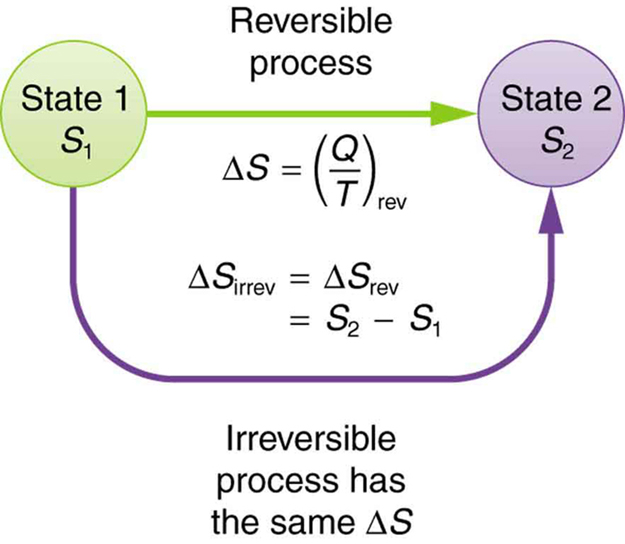Change in Entropy: When a system goes from state one to state two, its entropy changes by the same amount ΔS, whether a hypothetical reversible path is followed or a real irreversible path is taken.

### Example

Now let us take a look at the change in entropy of a Carnot engine and its heat reservoirs for one full cycle. The hot reservoir has a loss of entropy ΔSh=−Qh/Th, because heat transfer occurs out of it (remember that when heat transfers out, then Q has a negative sign). The cold reservoir has a gain of entropy ΔSc=Qc/Tc, because heat transfer occurs into it. (We assume the reservoirs are sufficiently large that their temperatures are constant. ) So the total change in entropy is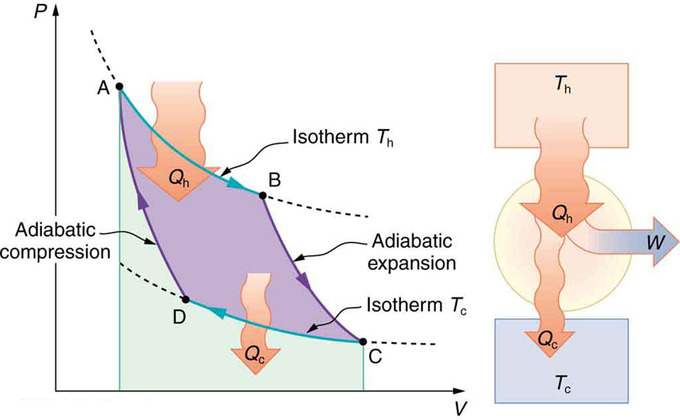PV Diagram for a Carnot Cycle: PV diagram for a Carnot cycle, employing only reversible isothermal and adiabatic processes. Heat transfer Qh occurs into the working substance during the isothermal path AB, which takes place at constant temperature Th. Heat transfer Qc occurs out of the working substance during the isothermal path CD, which takes place at constant temperature Tc. The net work output W equals the area inside the path ABCDA. Also shown is a schematic of a Carnot engine operating between hot and cold reservoirs at temperatures Th and Tc.

$\Delta \text{S}_{\text{tot}} = \Delta \text{S}_\text{h} + \Delta \text{S}_\text{c}$.

Thus, since we know that Qh/Th=Qc/Tc for a Carnot engine,

$\Delta \text{S}_{\text{tot}} = -\frac{\text{Q}_\text{h}}{\text{T}_\text{h}} + \frac{\text{Q}_\text{c}}{\text{T}_\text{c}} = 0$.

This result, which has general validity, means that the total change in entropy for a system in any reversible process is zero.

## Stastical Interpretation of Entropy

According the second law of thermodynamics, disorder is vastly more likely than order.

### Learning Objectives

Calculate the number of microstates for simple configurations

### Key Takeaways

#### Key Points

• Each microstate is equally probable in the example of coin toss. However, as a macrostate, there is a strong tendency for the most disordered state to occur.
• When tossing 100 coins, if the coins are tossed once each second, you could expect to get either all 100 heads or all 100 tails once in 2×1022 years.
• Molecules in a gas follow the Maxwell-Boltzmann distribution of speeds in random directions, which is the most disorderly and least structured condition out of all the possibilities.

#### Key Terms

• disorder: Absence of some symmetry or correlation in a many-particle system.
• Maxwell-Boltzmann distribution: A distribution describing particle speeds in gases, where the particles move freely without interacting with one another, except for very brief elastic collisions in which they may exchange momentum and kinetic energy.

The various ways of formulating the second law of thermodynamics tell what happens rather than why it happens. Why should heat transfer occur only from hot to cold? Why should the universe become increasingly disorderly? The answer is that it is a matter of overwhelming probability. Disorder is simply vastly more likely than order. To illustrate this fact, we will examine some random processes, starting with coin tosses.

### Coin Tosses

What are the possible outcomes of tossing 5 coins? Each coin can land either heads or tails. On the large scale, we are concerned only with the total heads and tails and not with the order in which heads and tails appear. The following table shows all possibilities along with numbers of possible configurations (or microstate; a detailed description of every element of a system). For example, 4 heads and 1 tail instance may occur on 5 different configurations, with any one of the 5 coins showing tail and all the rest heads. (HHHHT, HHHTH, HHTHH, HTHHH, THHHH)

• 5 heads, 0 tails: 1 microstate
• 4 heads, 1 tail: 5 microstates
• 3 heads, 2 tails: 10 microstates
• 2 heads, 3 tails: 10 microstates
• 1 head, 4 tails: 5 microstates
• 0 head, 5 tails: 1 microstate

Note that all of these conclusions are based on the crucial assumption that each microstate is equally probable. Otherwise, the analysis will be erroneous.

The two most orderly possibilities are 5 heads or 5 tails. (They are more structured than the others. ) They are also the least likely, only 2 out of 32 possibilities. The most disorderly possibilities are 3 heads and 2 tails and its reverse. (They are the least structured. ) The most disorderly possibilities are also the most likely, with 20 out of 32 possibilities for the 3 heads and 2 tails and its reverse. If we start with an orderly array like 5 heads and toss the coins, it is very likely that we will get a less orderly array as a result, since 30 out of the 32 possibilities are less orderly. So even if you start with an orderly state, there is a strong tendency to go from order to disorder, from low entropy to high entropy. The reverse can happen, but it is unlikely.

This result becomes dramatic for larger systems. Consider what happens if you have 100 coins instead of just 5. The most orderly arrangements (most structured) are 100 heads or 100 tails. The least orderly (least structured) is that of 50 heads and 50 tails. There is only 1 way (1 microstate) to get the most orderly arrangement of 100 heads. The total number of different ways 100 coins can be tossed—is an impressively large 1.27×1030. Now, if we start with an orderly macrostate like 100 heads and toss the coins, there is a virtual certainty that we will get a less orderly macrostate. If you tossed the coins once each second, you could expect to get either 100 heads or 100 tails once in 2×1022 years! In contrast, there is an 8% chance of getting 50 heads, a 73% chance of getting from 45 to 55 heads, and a 96% chance of getting from 40 to 60 heads. Disorder is highly likely.

### Real Gas

The fantastic growth in the odds favoring disorder that we see in going from 5 to 100 coins continues as the number of entities in the system increases. In a volume of 1 m3, roughly 1023 molecules (or the order of magnitude of Avogadro’s number) are present in a gas. The most likely conditions (or macrostate) for the gas are those we see all the time—a random distribution of atoms in space with a Maxwell-Boltzmann distribution of speeds in random directions, as predicted by kinetic theory as shown in (a). This is the most disorderly and least structured condition we can imagine.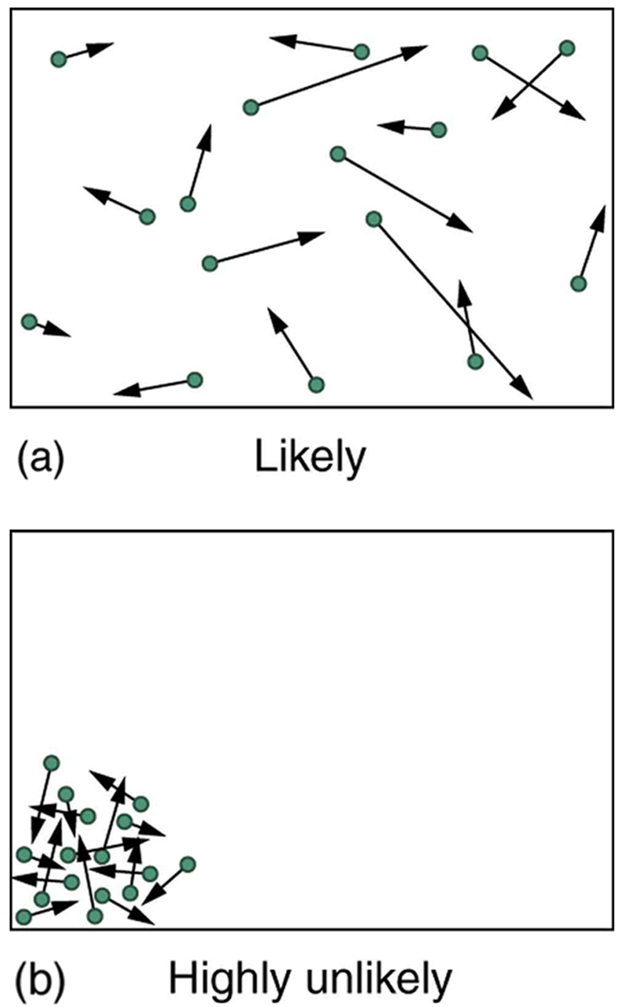Kinetic Theory: (a) The ordinary state of gas in a container is a disorderly, random distribution of atoms or molecules with a Maxwell-Boltzmann distribution of speeds. It is so unlikely that these atoms or molecules would ever end up in one corner of the container that it might as well be impossible. (b) With energy transfer, the gas can be forced into one corner and its entropy greatly reduced. But left alone, it will spontaneously increase its entropy and return to the normal conditions, because they are immensely more likely.

In contrast, one type of very orderly and structured macrostate has all of the atoms in one corner of a container with identical velocities. There are very few ways to accomplish this (very few microstates corresponding to it), and so it is exceedingly unlikely ever to occur. (See (b). ) Indeed, it is so unlikely that we have a law saying that it is impossible, which has never been observed to be violated—the second law of thermodynamics.

## Order to Disorder

Entropy is a measure of disorder, so increased entropy means more disorder in the system.

### Learning Objectives

Discuss entropy and disorder within a system

### Key Takeaways

#### Key Points

• Mixing two systems may decrease the entropy of one system, but increase the entropy of the other system by a greater amount, producing an overall increase in entropy.
• After mixing water at two different temperatures, the energy in the system that could have been used to run a heat engine is now unavailable to do work. Also, the process made the whole system more less structured.
• Entropy, unavailability of energy, and disorder are not only related but are in fact essentially equivalent.

#### Key Terms

• entropy: A measure of how evenly energy (or some analogous property) is distributed in a system.
• disorder: Absence of some symmetry or correlation in a many-particle system.

Entropy is a measure of disorder. This notion was initially postulated by Ludwig Boltzmann in the 1800s. For example, melting a block of ice means taking a highly structured and orderly system of water molecules and converting it into a disorderly liquid in which molecules have no fixed positions. There is a large increase in entropy in the process.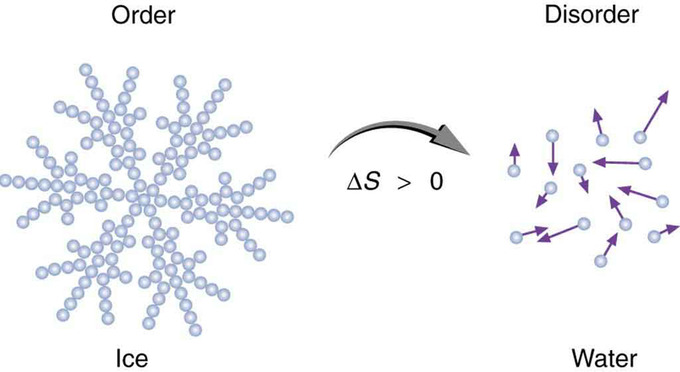Entropy of Ice: When ice melts, it becomes more disordered and less structured. The systematic arrangement of molecules in a crystal structure is replaced by a more random and less orderly movement of molecules without fixed locations or orientations. Its entropy increases because heat transfer occurs into it. Entropy is a measure of disorder.

### Example

As an example, suppose we mix equal masses of water originally at two different temperatures, say 20.0º C and 40.0º C. The result is water at an intermediate temperature of 30.0º C. Three outcomes have resulted:

1. Entropy has increased.
2. Some energy has become unavailable to do work.
3. The system has become less orderly.

### Entropy, Energy, and Disorder

Let us think about each of the results. First, entropy has increased for the same reason that it did in the example above. Mixing the two bodies of water has the same effect as heat transfer from the hot one and the same heat transfer into the cold one. The mixing decreases the entropy of the hot water but increases the entropy of the cold water by a greater amount, producing an overall increase in entropy.

Second, once the two masses of water are mixed, there is only one temperature—you cannot run a heat engine with them. The energy that could have been used to run a heat engine is now unavailable to do work.

Third, the mixture is less orderly, or to use another term, less structured. Rather than having two masses at different temperatures and with different distributions of molecular speeds, we now have a single mass with a uniform temperature.

These three results—entropy, unavailability of energy, and disorder—are not only related but are in fact essentially equivalent.

## Heat Death

The entropy of the universe is constantly increasing and is destined for thermodynamic equilibrium, called the heat death of the universe.

### Learning Objectives

Describe processes that lead to the heat death of the universe

### Key Takeaways

#### Key Points

• In the early, energetic universe, all matter and energy were easily interchangeable and identical in nature.
• As entropy increases, less and less energy in the universe is available to do work.
• The universe is destined for thermodynamic equilibrium —maximum entropy. This is often called the heat death of the universe, and will mean the end of all activity.

#### Key Terms

• geothermal: Pertaining to heat energy extracted from reservoirs in the Earth’s interior.
• entropy: A measure of how evenly energy (or some analogous property) is distributed in a system.
• asteroid: A naturally occurring solid object, which is smaller than a planet and is not a comet, that orbits a star.

In the early, energetic universe, all matter and energy were easily interchangeable and identical in nature. Gravity played a vital role in the young universe. Although it may have seemed disorderly, there was enormous potential energy available to do work—all the future energy in the universe.

As the universe matured, temperature differences arose, which created more opportunity for work. Stars are hotter than planets, for example, which are warmer than icy asteroids, which are warmer still than the vacuum of the space between them. Most of these are cooling down from their usually violent births, at which time they were provided with energy of their own—nuclear energy in the case of stars, volcanic energy on Earth and other planets, and so on. Without additional energy input, however, their days are numbered.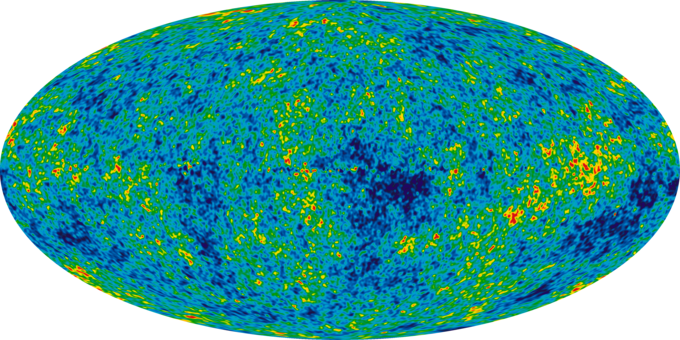Infant Universe: The image of an infant universe reveals temperature fluctuations (shown as color differences) that correspond to the seeds that grew to become the galaxies.

As entropy increases, less and less energy in the universe is available to do work. On Earth, we still have great stores of energy such as fossil and nuclear fuels; large-scale temperature differences, which can provide wind energy; geothermal energies due to differences in temperature in Earth’s layers; and tidal energies owing to our abundance of liquid water. As these are used, a certain fraction of the energy they contain can never be converted into doing work. Eventually, all fuels will be exhausted, all temperatures will equalize, and it will be impossible for heat engines to function, or for work to be done.

Since the universe is a closed system, the entropy of the universe is constantly increasing, and so the availability of energy to do work is constantly decreasing. Eventually, when all stars have died, all forms of potential energy have been utilized, and all temperatures have equalized (depending on the mass of the universe, either at a very high temperature following a universal contraction, or a very low one, just before all activity ceases) there will be no possibility of doing work.

Either way, the universe is destined for thermodynamic equilibrium—maximum entropy. This is often called the heat death of the universe, and will mean the end of all activity. However, whether the universe contracts and heats up, or continues to expand and cools down, the end is not near. Calculations of black holes suggest that entropy can easily continue for at least 10100 years.

## Living Systems and Evolution

It is possible for the entropy of one part of the universe to decrease, provided the total change in entropy of the universe increases.

### Learning Objectives

Formulate conditions that allow decrease of the entropy in one part of the universe

### Key Takeaways

#### Key Points

• Living organisms have evolved to be highly structured, and much lower in entropy than the substances from which they grow.
• It possible for a system to decrease its entropy provided the total change in entropy of the universe increases: $\Delta \text{S}_{\text{tot}} = \Delta \text{S}_{\text{sys}} + \Delta \text{S}_{\text{env}} >0$.
• The Earth can be viewed as a heat engine operating between a hot reservoir supplied by the Sun and a cold reservoir supplied by dark outer space.

#### Key Terms

• entropy: A measure of how evenly energy (or some analogous property) is distributed in a system.

Some people misunderstand the second law of thermodynamics, stated in terms of entropy, to say that the process of the evolution of life violates this law. Over time, complex organisms evolved from much simpler ancestors, representing a large decrease in entropy of the Earth’s biosphere. It is a fact that living organisms have evolved to be highly structured, and much lower in entropy than the substances from which they grow. But it is always possible for the entropy of one part of the universe to decrease, provided the total change in entropy of the universe increases. In equation form, we can write this as

$\Delta \text{S}_{\text{tot}} = \Delta \text{S}_{\text{sys}} + \Delta \text{S}_{\text{env}} >0$.

Thus ΔSsys can be negative as long as ΔSenv is positive and greater in magnitude.

How is it possible for a system to decrease its entropy? Energy transfer is necessary. If I gather iron ore from the ground and convert it into steel and build a bridge, my work (and used energy) has decreased the entropy of that system. Energy coming from the Sun can decrease the entropy of local systems on Earth—that is, ΔSsys is negative. But the overall entropy of the rest of the universe increases by a greater amount—that is, ΔSenv is positive and greater in magnitude. Thus, ΔStot>0, and the second law of thermodynamics is not violated.

Every time a plant stores some solar energy in the form of chemical potential energy, or an updraft of warm air lifts a soaring bird, the Earth can be viewed as a heat engine operating between a hot reservoir supplied by the Sun and a cold reservoir supplied by dark outer space—a heat engine of high complexity, causing local decreases in entropy as it uses part of the heat transfer from the Sun into deep space. However, there is a large total increase in entropy resulting from this massive heat transfer. A small part of this heat transfer is stored in structured systems on Earth, producing much smaller local decreases in entropy.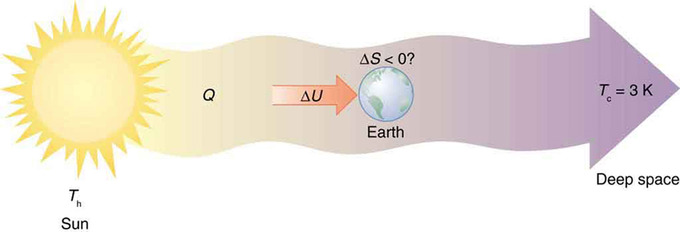Earth’s Entropy: Earth’s entropy may decrease in the process of intercepting a small part of the heat transfer from the Sun into deep space. Entropy for the entire process increases greatly while Earth becomes more structured with living systems and stored energy in various forms.

## Global Warming Revisited

The Second Law of Thermodynamics may help provide explanation for the global warming over the last 250 years.

### Learning Objectives

Describe effect of the heat dumped into the environment on the Earth’s atmospheric temperature

### Key Takeaways

#### Key Points

• As heat engines operate, a great deal of heat is lost to the environment due to inefficiencies.
• Even in a Carnot engine, which is the most efficient theoretical engine, there is a heat loss determined by the ratio of temperature of the engine and its environment.
• As more heat is dumped into the environment, Earth’s atmospheric temperature will increase.

#### Key Terms

• Carnot cycle: A theoretical thermodynamic cycle. It is the most efficient cycle for converting a given amount of thermal energy into work.
• absolute zero: The coldest possible temperature: zero on the Kelvin scale and approximately -273.15°C and -459.67°F. The total absence of heat; the temperature at which motion of all molecules would cease.

The Second Law of Thermodynamics may help provide explanation for why there have been increases in Earth’s temperatures over the last 250 years (often called “Global Warming”), and many professionals are concerned that the entropy increase of the universe is a real threat to the environment.Global Land-Ocean Temperature: Global mean land-ocean temperature change from 1880 – 2012, relative to the 1951 – 1980 mean. The black line is the annual mean and the red line is the five-year running mean. The green bars show uncertainty estimates.

As an engine operates, heat flows from a heat tank of greater temperature to a heat sink of lesser temperature. In between these states, the heat flow is turned into useful energy with the help of heat engines. As these engines operate, however, a great deal of heat is lost to the environment due to inefficiencies. In a Carnot engine, which is the most efficient theoretical engine based on Carnot cycle, the maximum efficiency is equal to one minus the temperature of the heat sink (Tc) divided by the temperature of the heat source (Th).

$(\text{Eff}_\text{c} = 1 - \frac{\text{T}_\text{c}}{\text{T}_\text{h}})$.

This ratio shows that for a greater efficiency to be achieved there needs to be the greatest difference in temperature available. This brings up two important points: optimized heat sinks are at absolute zero, and the longer engines dump heat into an isolated system the less efficient engines will become.

Unfortunately for engine efficiency, day-to-day life never operates in absolute zero. In an average car engine, only 14% to 26% of the fuel which is put in is actually used to make the car move forward. This means that 74% to 86% is lost heat or used to power accessories. According to the U.S. Department of Energy, 70% to 72% of heat produced by burning fuel is heat lost by the engine. The excess heat lost by the engine is then released into the heat sink, which in the case of many modern engines would be the Earth’s atmosphere. As more heat is dumped into the environment, Earth’s atmospheric (or heat sink) temperature will increase. With the entropy of the environment constantly increasing, searching for new, more efficient technologies and new non-heat engines has become a priority.

## Thermal Pollution

Thermal pollution is the degradation of water quality by any process that changes ambient water temperature.

### Learning Objectives

Identify factors that lead to thermal pollution and its ecological effects

### Key Takeaways

#### Key Points

• All heat engines require heat transfer, achieved by providing (and maintaining) temperature difference between engine’s heat source and heat sink. Cooling water is typically used to maintain the temperature difference.
• Elevated water temperature typically decreases the level of dissolved oxygen of water, affecting ecosystem composition.
• Cooling heated water is not a solution for thermal pollution because extra work is required for the cooling, leading to more heat exhaust into the environment.

#### Key Terms

• heat engine: Any device which converts heat energy into mechanical work.
• heat pump: A device that transfers heat from something at a lower temperature to something at a higher temperature by doing work.

Thermal pollution is the degradation of water quality by any process that changes ambient water temperature. A common cause of thermal pollution is the use of water as a coolant, for example, by power plants and industrial manufacturers. When water used as a coolant is returned to the natural environment at a higher temperature, the change in temperature decreases oxygen supply, and affects ecosystem composition.

As we learned in our Atom on “Heat Engines”, all heat engines require heat transfer, achieved by providing (and maintaining) temperature difference between engine’s heat source and heat sink. Water, with its high heat capacity, works extremely well as a coolant. But this means that cooling water should be constantly replenished to maintain its cooling capacity.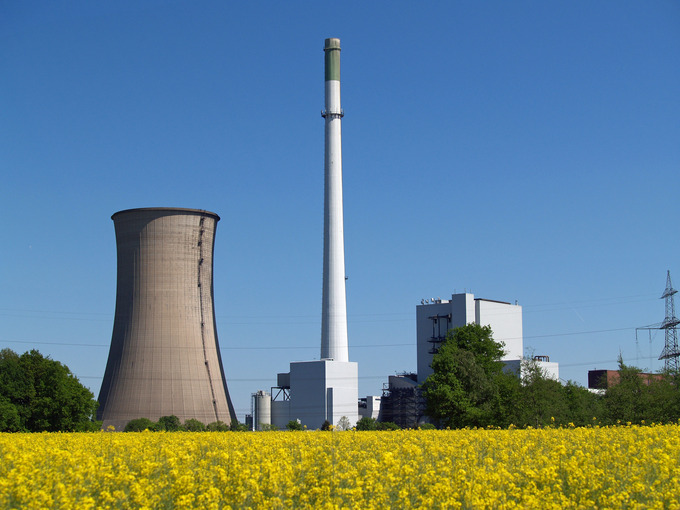Cooling Tower: This is a cooling tower at Gustav Knepper Power Station, Dortmund, Germany. Cooling water is circulated inside the tower.

### Ecological Effects

Elevated water temperature typically decreases the level of dissolved oxygen of water. This can harm aquatic animals such as fish, amphibians, and other aquatic organisms. An increased metabolic rate may result in fewer resources; the more adapted organisms moving in may have an advantage over organisms that are not used to the warmer temperature. As a result, food chains of the old and new environments may be compromised. Some fish species will avoid stream segments or coastal areas adjacent to a thermal discharge. Biodiversity can decrease as a result. Many aquatic species will also fail to reproduce at elevated temperatures.

Some may assume that by cooling the heated water, we can possibly fix the issue of thermal pollution. However, as we noted in our previous Atom on “Heat Pumps and Refrigerators”, work required for the additional cooling leads to more heat exhaust into the environment. Therefore, it makes the situation even worse.